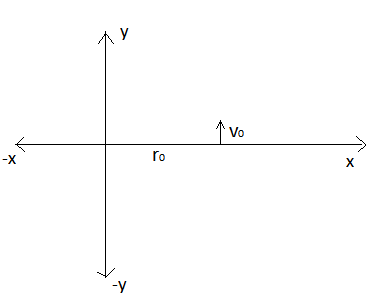# Solve for trajectory againWe have placed a particle of mass $m$ at $\overset { \rightarrow }{ r } ={ r }_{ 0 }\hat { i }$.

At $t=0$ we have given it a velocity of $\overrightarrow { v } ={ v }_{ 0 }\hat { j }$.In the whole co-ordinate plane the force is given by $\overrightarrow{F}=-\overrightarrow{r}Newton$

The title says solve for trajectory but I am telling you that the trajectory of the particle is ellipse.If the eccentricity of the ellipse is given by $e=\frac {\sqrt {a} }{ b }$ where $a,b$ are co-prime integers and a is square free then find $a\times b$

Details and Assumptions

1)${ v }_{ 0 }=2 m/s,{ r }_{ 0 }=1 metre,m=1Kg$

2)There are no other force acting on the particle except the force given.

3) Ignore gravity

×

Problem Loading...

Note Loading...

Set Loading...